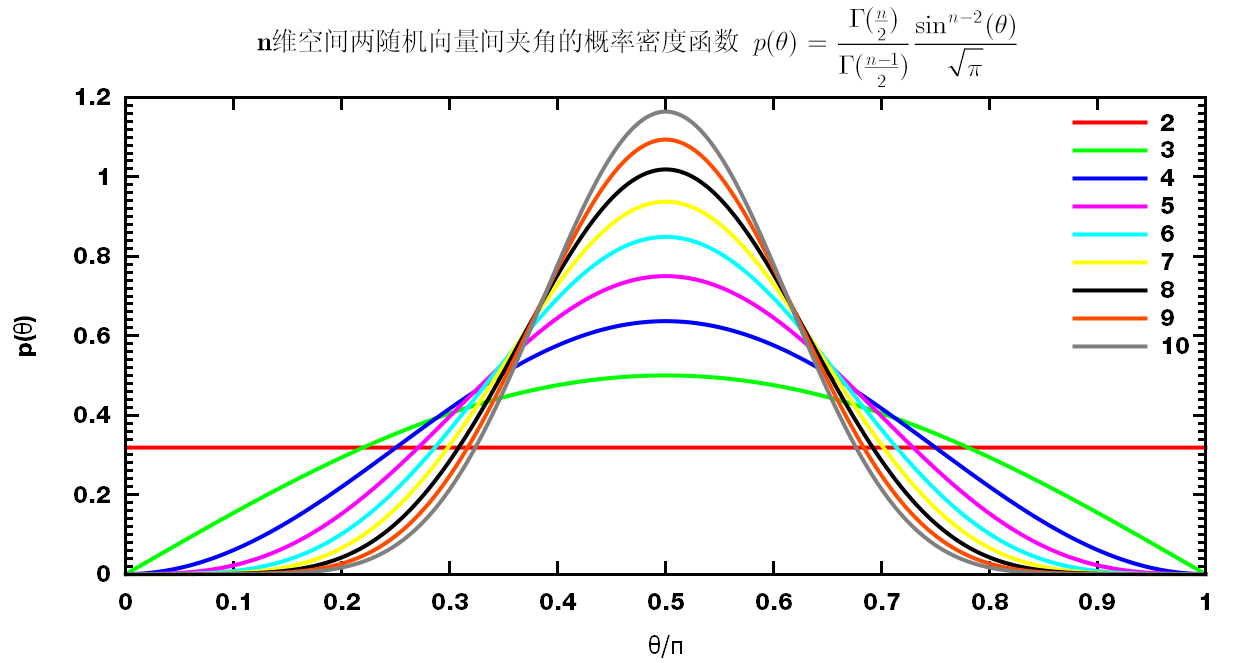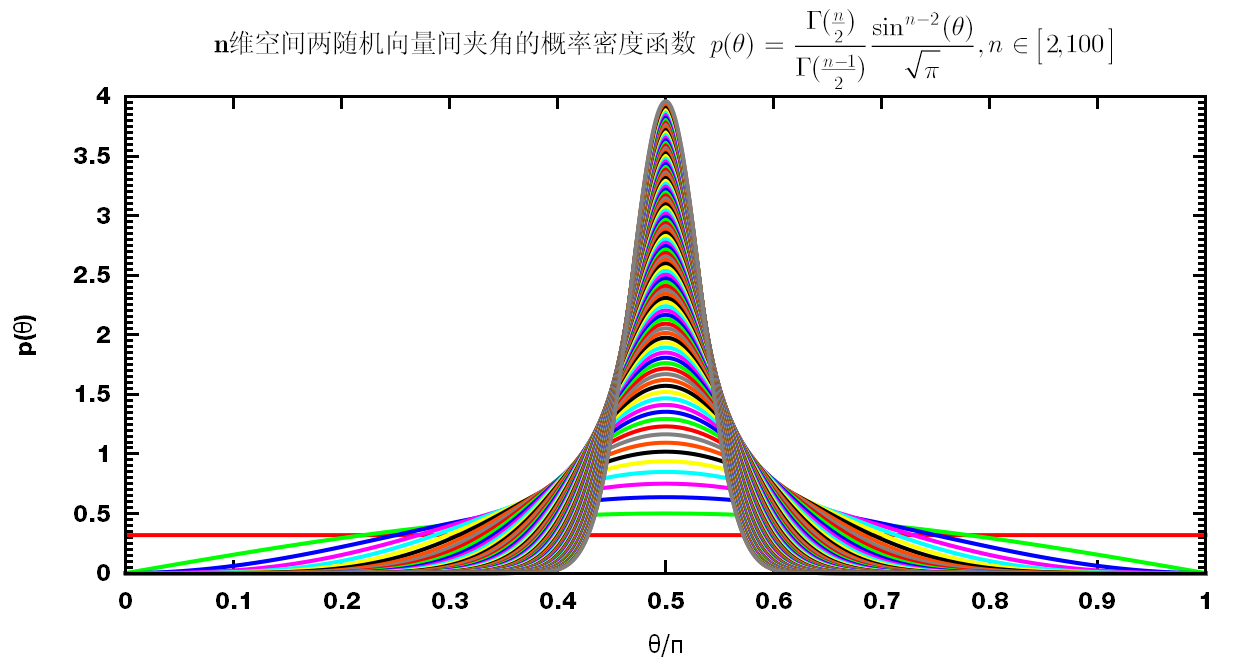# 空间中两随机向量间夹角的概率密度分布

## 2013-03-18 10:26:43

2014-07-12 17:52:11 补充

$p(\theta)= \frac{\Gamma(\frac{n}{2})}{\Gamma(\frac{n-1}{2})} \frac{\sin^{n-2}(\theta)}{\sqrt\pi}$

$S_{n-1}={\rmd {V_n} \over \rmd R}={2\p^{n/2} \over \G(n/2)} R^{n-1}$

$p(\theta)\rmd \theta={\Delta S \over S_{n-1} }={S_{n-2}(R\sin\theta)R \rmd \theta \over S_{n-1}(R)}$$\left\vert p(x) \rmd x \right\vert= \left\vert p(y) \rmd y\right\vert$

$p(y)=p(x) \left\vert {\rmd x \over \rmd y} \right\vert = p(x){1 \over \left\vert \rmd y/\rmd x \right\vert}$

$\alg \langle f(\cos\theta) \rangle &=\int_0^\pi f(\cos\theta)p(\theta)\rmd \theta \\ &={1 \over 2}\int_0^\pi f(\cos\theta)\sin\theta \rmd\theta \\ &={1 \over 2}\int_{-1}^1 f(\cos\theta)\rmd{\cos\theta} \\ &={1 \over 2}\int_{-1}^1 f(x)\rmd x \ealg$

$\langle \cos^m\theta \rangle=\begin{cases} 0 , &m=2k+1 \\ {1 \over m+1 }, &m=2k \end{cases}$

1. 中文维基, n维球面
2. 百度贴吧, n维空间中两个随机向量夹角的概率密度函数
 随意赞赏微信支付宝
◆本文地址: , 转载请注明◆
◆评论问题: https://jerkwin.herokuapp.com/category/3/博客, 欢迎留言◆访问人次(2017年1月27日起): | 最后更新: 2021-04-22 10:35:31 CST | 版权所有 © 2008 - 2021 Jerkwin